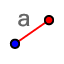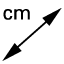# Triangle Congruence: ASA

## Construct a triangle with a 37° angle and a 73° angle connected by a side of length 4.

1. Select the SEGMENT WITH GIVEN LENGTHtool, and enter a length of 4. 2. Select the LINEtool. Click on point A and then somewhere above or below segment AB.﻿ 3. Select the ANGLEtool. Click points B, A, and C to measure angle A. If the measurement is over 180°, undo and click on them in reverse order. 4. Select the MOVEtool, and move point C until angle BAC has a measure of 37°. 5. Repeat steps 2–4 to create angle DBA with a measure of 73°. 6. The place where the lines meet is the third vertex of your triangle. Use the INTERSECTtool to mark it as point E. 7. Select the DISTANCE OR LENGTHtool, and then click on points A and E to measure the distance between them. Do the same for points E and B. 8. Answer the questions below the applet by constructing each triangle describe below.

Construct a triangle with a 37° angle and a 73° angle connected by a side of length 4. What are the lengths of the other two sides?

Construct a triangle with a 12° angle and a 53° angle connected by a side of length 5. What are the lengths of the other two sides?

## Convert an AAS problem into an ASA problem by first finding the third angle.

Construct a triangle with a 103° angle and a 28° angle, with a side of length 8 between the 28° angle and third angle. What are the lengths of the other two sides?